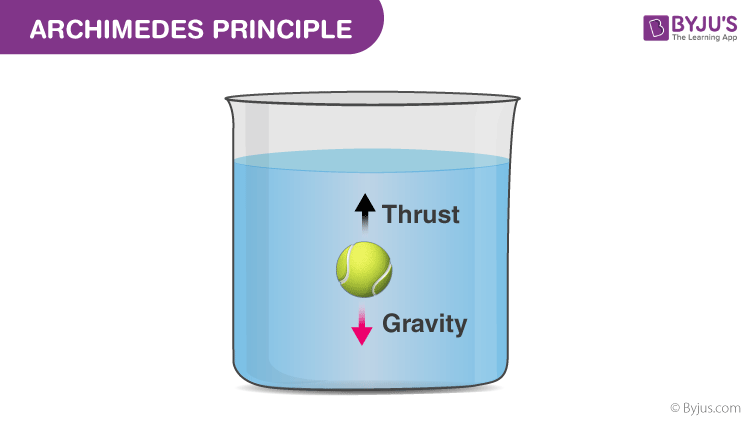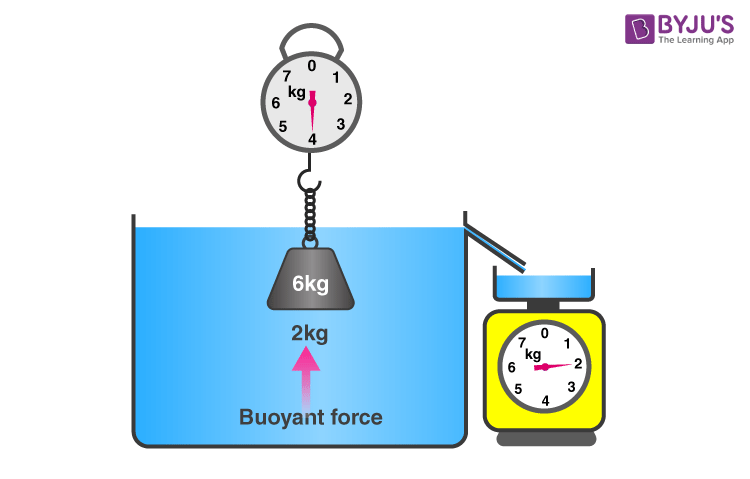# Archimedes Principle

Archimedes’ principle deals with the forces applied to an object by fluids surrounding it. This applied force reduces the net weight of the object submerged in a fluid. In this article, let us familiarize ourselves with Archimedes’ principle.

## What is the Archimedes’ Principle?

Archimedes’ principle states that:

“The upward buoyant force that is exerted on a body immersed in a fluid, whether partially or fully submerged, is equal to the weight of the fluid that the body displaces and acts in the upward direction at the center of mass of the displaced fluid”.

The value of thrust force is given by the Archimedes law which Archimedes of Syracuse of Greece discovered. When an object is partially or fully immersed in a liquid, the apparent loss of weight is equal to the weight of the liquid displaced by it.

### Archimedes’ Principle ExplanationIf you look at the figure, the weight due to gravity is opposed by the thrust provided by the fluid. The object inside the liquid only feels the total force acting on it as the weight. Because the actual gravitational force is decreased by the liquid’s upthrust, the object feels as though its weight is reduced. The apparent weight is thus given by:

Apparent weight= Weight of object (in the air) – Thrust force (buoyancy)

Archimedes’ principle tells us that the weight loss is equal to the weight of liquid the object displaces.

Watch the video to visualize Archimedes’ Principle with the help of animations!### Archimedes’ Principle Formula

In simple form, the Archimedes law states that the buoyant force on an object is equal to the weight of the fluid displaced by the object. Mathematically written as:

 Fb = ρ x g x V

Where Fb is the buoyant force, ρ is the density of the fluid, V is the submerged volume, and g is the acceleration due to gravity.

### Archimedes’ Principle Derivation

We know that the density is defined as

$$\begin{array}{l}Density\,(\rho )=\frac{Mass\,(M)}{Volume\,(V)}\end{array}$$

Therefore, the mass of the displaced liquid can be written as follows:

$$\begin{array}{l}Mass\,(M)=Density\,(\rho ){Volume\,(V)}\end{array}$$

Now, the weight of the displaced liquid can be calculated as follows:

$$\begin{array}{l}Weight=Mass\times Acceleration\,due\,to\,gravity\end{array}$$

$$\begin{array}{l}Weight=Mass\times g=\rho \times V\times g\end{array}$$

From Archimedes’ principle, we know that the apparent loss of weight is equal to the weight of the water displaced therefore the thrust force is given by the following equation:

$$\begin{array}{l}Thrust\,Force=\rho \times V\times g\end{array}$$

Where ρ is the density of the liquid, V is the volume of liquid displaced and g is the acceleration due to gravity.
The thrust force is also called the buoyant force because it is responsible for objects floating. Thus, this equation is also called the law of buoyancy.

### Archimedes’ Principle Examples

Q1. Calculate the resulting force, if a steel ball of radius 6 cm is immersed in water.

Ans: Given,

Radius of steel ball = 6 cm = 0.06 m

Volume of steel ball,

$$\begin{array}{l}V = \frac{4}{3}\pi r^{3}\end{array}$$
$$\begin{array}{l}V = \frac{4}{3}\pi 0.06^{3}\end{array}$$

∴V = 9.05 × 10-4 m3

Density of water, ρ = 1000 kg.m-3

Acceleration due to gravity, g = 9.8 m.s-2

From Archimedes’ principle formula,

Fb = ρ × g × V

F= (1000 kg.m-3)(9.8 m.s-2)(9.05 × 10-4 m3)

∴Fb = 8.87 N

Q2. Calculate the buoyant force, if a floating body is 95% submerged in water. The density of water is 1000 kg.m-3.

Ans: Given,
Density of water, p = 1000 kg.m-3
From Archimedes’ principle formula,
Fb = ρ × g × V

or

Vb × ρb × g = ρ × g × V

Where,

ρ,g, and V are the density, acceleration due to gravity, and volume of the water

Vb, ρb, and g are the volume, density, and acceleration due to gravity of body immersed

Rearranging the equation,

$$\begin{array}{l}\rho _{b}=\frac{V\rho }{V_{b}}\end{array}$$

Since 95% of the body is immersed,

0.95 × V= V

∴ρ= 950 kg.m-3

## Archimedes’ Principle Experiment• Take a container filled with water to the brim.
• Now take any solid object you like and measure its weight using a spring balance. Note this down.
• Keep the object attached to the spring balance and submerge it in the water. Just make sure the spring balance is not submerged.
• Now, note down the weight shown by the spring balance. You will notice that it is less. Some water will be displaced into the bowl.
• Collect this water and weigh it. You will find that the weight of the water will be exactly equal to the loss of weight of the object!

## Archimedes’ Principle Applications

Following are the applications of Archimedes’ principle:

### Submarine:

The reason why submarines are always underwater is that they have a component called ballast tank which allows the water to enter making the submarine be in its position underwater as the weight of the submarine is greater than the buoyant force.

### Hot-air balloon:

The reason why hot-air balloons rise and float in mid-air is because the buoyant force of the hot-air balloon is less than the surrounding air. When the buoyant force of the hot-air balloon is more, it starts to descend. This is done by varying the quantity of hot air in the balloon.

### Hydrometer:

A hydrometer is an instrument used for measuring the relative density of liquids. Hydrometer consists of lead shots which makes them float vertically on the liquid. The lower the hydrometer sinks, the lesser is the density of the liquid.

## Frequently Asked Questions – FAQs

Q1

### What does Archimedes Principle state?

Archimedes’ principle states that an object submerged in a fluid, fully or partially, experiences an upward buoyant force that is equal in magnitude to the force of gravity on the displaced fluid.

Q2

### Who discovered the Archimedes’ Principle?

Greek mathematician Archimedes discovered the Archimedes’ principle.

Q3

### How does the Archimedes’ principle apply to ships?

Archimedes continued to do more experiments and came up with a buoyancy principle that a ship will float when the weight of the water it displaces equals the weight of the ship and anything will float if it is shaped to displace its own weight of water before it reaches the point where it will submerge.

Q4

### Where is the Archimedes’ principle used?

Archimedes’ principle is used in the design principle of ships and submarines. Hydrometers are based on the principle of Archimedes.

Q5

### How can the Archimedes’ Principle be used to determine the density?

The weight of the fluid displaced is equal to the buoyant force on a submerged object. The mass divided by the volume thus determined gives a measure of the average density of the object.

Stay tuned with BYJU’S to learn more interesting topics with engaging videos!

Test your knowledge on Archimedes Principle